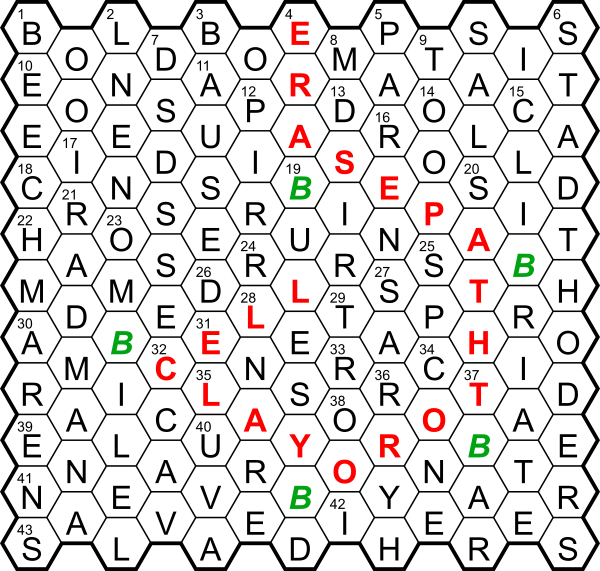## Queen by Rebel

### Puzzle explanation

The letters omitted from across answers spelt out “way in top mid, bees deflecting”. Starting downward from central cell in the top row and turning when hitting a B traced the message “erase path to royal cell” as shown here. The letters omitted from down answers spelt out “title bee; core occupiers”, leading to the phrase QUEEN BEE and its initials QB to be entered in the central cell.

### Clue explanations

Conventions: * = anagram, < = reversal

Across
1 BOLD BO(W) B + OLD BO(y)
3 BORD(A)R ORD in BR
4 EMPT(Y)SIS MISSTEP *
8 MA(I)OL(I)CA (A + CLOAM) <
9 TAI(N)T TAIT
10 E(T)ON SUI(T) EONS + U + I
12 PASE(O) APES *
16 (P)ROSIT (’TIS + OR) <
17 (M)INDS IN + D(ungenes)S
18 CROS(I)ER SCORER *
22 HAME(D) HAM + E
24 (B)RUIN (f)RUI(t) + N
25 ST(E)R(E)O STRONG − NG
26 D(EE)RL(E)TS DOLTS with R for O
30 AMICU(S) AM + ICU
31 (D)ENET (r)E(i)N(v)E(s)T(s)
33 RAPHID(E) RAP + HID
35 LAY-O(FF) LAY + O’
36 ROTA(L) R(un) O(ff) T(he) A(genda)
38 ORNAT(E)R OR + TAN < + ER
39 EN(C)LAVE LAV in ENE
40 (T)URBI(T)H (yo)U + R + HIB <
41 NAEV(I) EVAN *
42 I(N)HERES (HER + (Evi)E) in IS
43 SALVA(G)ED SAD around VEAL *
Down
1 BEECH MAR(T)ENS (BEEN + S) around (CH + MARE)
2 L(I)NEN L(eaves) + NE(ed) + N
5 PAREN(T) AR in PEN
7 D(E)S(EE)DS D(isk)S + D(isk)S
11 A(B)USED A + USED
12 PI(E)RR(E) (R(aise) + RIP) <
13 D(E)SIR(E) (Jes)S(ica) in DIR
14 (C)OOP (POOH − H) <
15 C(O)LIBRI C(a)L(l) I(f) B(i)R(d) I(s)
19 BU(R)LE(R)S BS around ULE
20 S(E)ATH (ha)S A TH(ing)